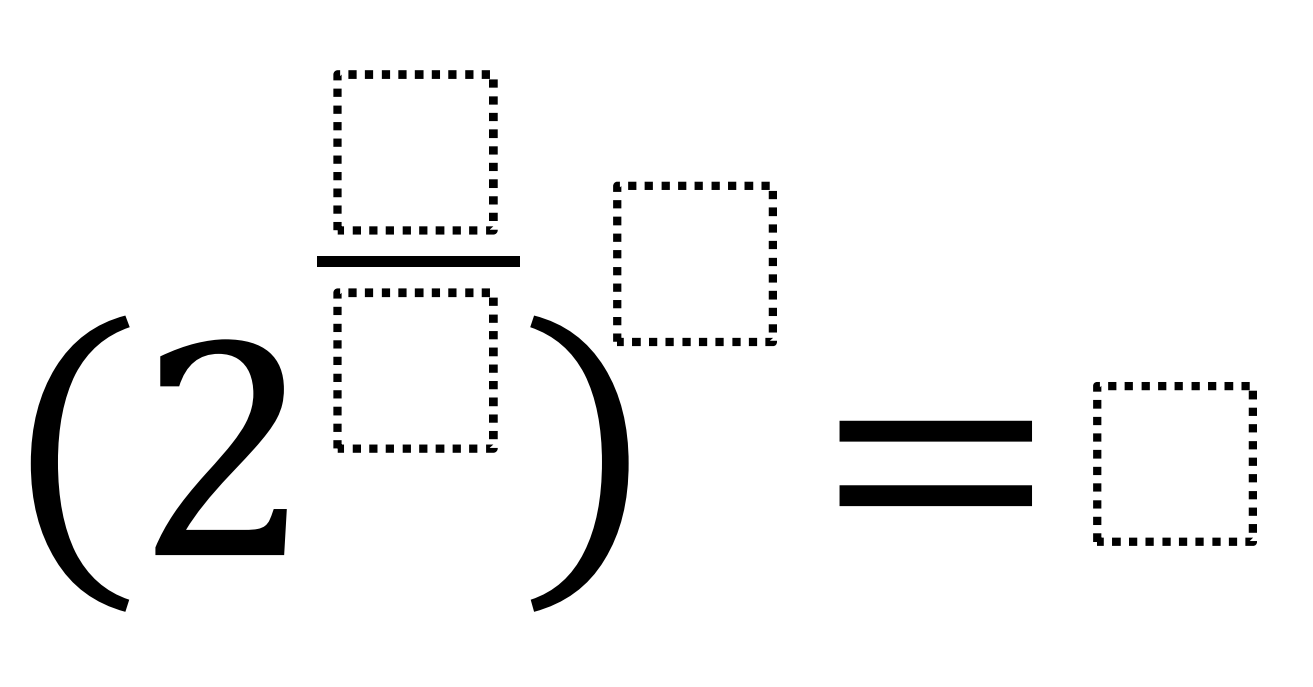# Fractional Power to a Power

Directions: Using the digits 1 to 9 at most once, fill in the boxes to create a true statement.### Hint

How are the fractional exponent and the exponent outside the parenthesis related?

### Answer

Several possible answers:

(2^1/3)^6 = 4 — the 6 and 1 can change places
(2^1/3)^9 = 8 — the 9 and 1 can change places
(2^6/2)^1 = 8 — the 1 and 6 can change places

Source: Kate Nerdypoo

## Properties of Exponents 4

Directions: Using the digits 1 to 9 at most one time each, fill in the …